Given the information on the diagram, determine the coordinates of point 101. This is a Distance-Distance intersection. Figure E-1Example Computation

 Step (1) Inverse along the base line from 30 to 20.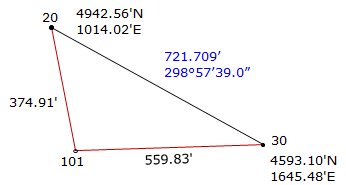Step (2) Compute the angle at 30 by Law of Cosines.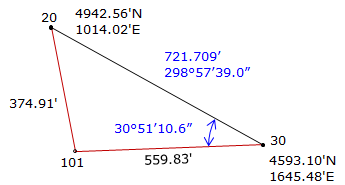Step (3) Compute the direction from 30 to 101.Step (4) Perfom a forward computation from 30 to 101.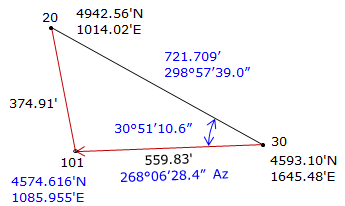Math check: Compute coordinates from point 20.

 Step (1) Compute angle at 20 by Law of Sines.Step (2) Compute direction from 201 to 101.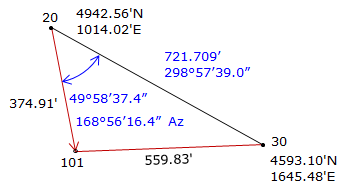Step (3) Perfom a forward computation from 20 to 101.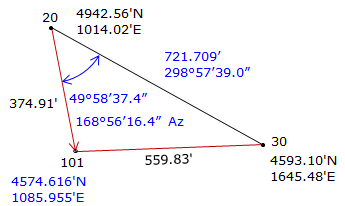From 30 From 20 North 4574.616 4574.616 √ East 1085.955 1085.955 √